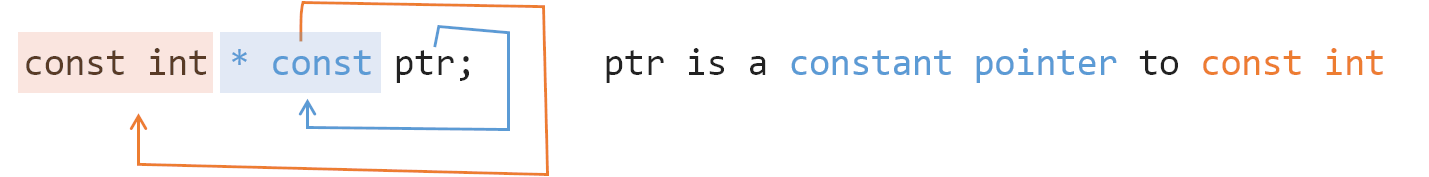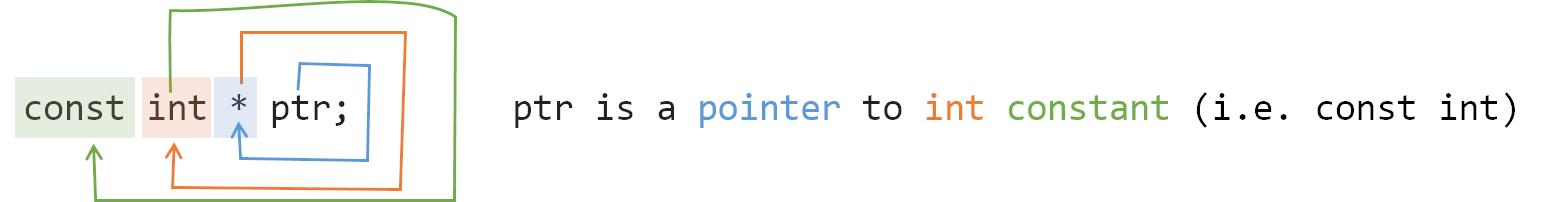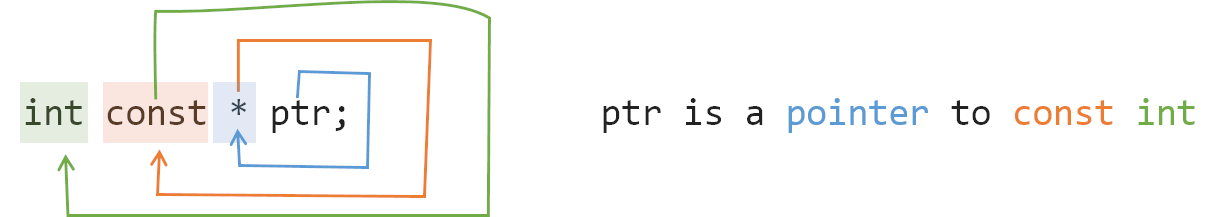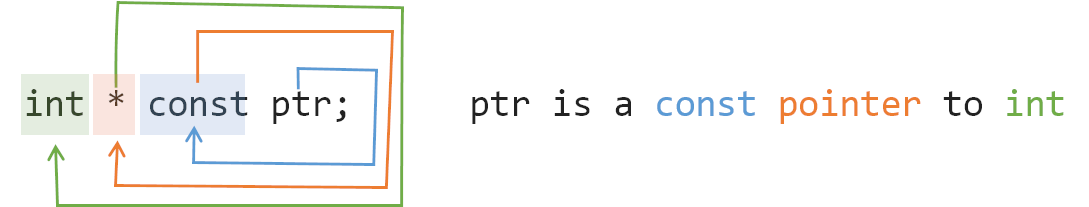When I was using C++ const, I was dizzy when I saw the usage of const, such as `const int*`, `const int * const`, `int const *`. And after C++ 11 added constexpr, I don’t know what the difference between it and const is. This article is mainly to organize the knowledge of this area.

## const

The general use of const is to modify variables, references, and pointers, after which they become constants. It should be noted that const does not distinguish between compile-time constants and run-time constants, and const only guarantees that they are not directly modified at run-time.

In the general case, const is placed on the left, indicating constants.

 ``````1 2 3 `````` ``````const int x = 100; //Constant const int& rx = x; //Constant references const int* px = &x;//Constant Pointer ``````

Adding const to a variable makes it a “constant”, which can only be read, but not written. The compiler will check for all writes to it and issue warnings to prevent intentional or unintentional changes at the compilation stage. In this way, const constants are relatively safe to use. So when you design a function, if you modify the arguments with const, you can ensure efficiency and safety.

In addition, const can be declared on member functions. Const is placed after the function to indicate that the function is a “constant” and that the execution of the function is const and does not modify the state of the object (i.e. member variables), e.g.

 `````` 1 2 3 4 5 6 7 8 9 10 11 12 13 14 `````` ``````class DemoClass final { private: const long MAX_SIZE = 256; // const member variable int m_value; // Member Variables public: int get_value() const // const member function { // error: assignment of member 'DemoClass::m_value' // in read-only object m_value = 100; return m_value; } }; ``````

There are other cases where const is used for pointers. const is placed on the leftmost side of the declaration and represents a pointer to a constant (pointer to const int), which points to a “read-only variable” and is not allowed to be modified.

 ``````1 2 3 `````` `````` int x = 100; const int* px = &x; *px = 102; // error: assignment of read-only ``````

const is placed to the right of “*” to indicate that the pointer is a constant (const pointer to int) and that the pointer cannot be modified, while the variable pointed to can be modified.

 ``````1 2 3 4 5 `````` `````` int x = 100; int b = 150; int* const px = &x; *px = 102; // success px = &b; // error: assignment of read-only location ``````

There is another is const placed on both sides of the “*”, indicating that the pointer and pointing to constants are pointers (const pointer to const int), indicating that both pointers and variables can not be modified, the specific you can go try. It is important to note that the following cases are equivalent.

• `const int *` == `int const *`
• `const int * const` == `int const * const`

For example, for this statement below.

 ``````1 2 3 4 5 6 7 `````` `````` +-------+ | +-+ | | ^ | | char *str; ^ ^ | | | +---+ | +-----------+ ``````
• The first thing that comes to mind is what is str? We turn clockwise with str, and the first thing we encounter is `[`, so that means str is an array, so str is an array with a capacity of 10.
• Then we move clockwise again, and the next one we encounter is `*`, so that means it’s a pointer, so str is a pointer to an array of capacity 10.
• We continue turning clockwise and find that we encounter the line terminator `;`, skip it and continue turning and find that we encounter `char`, so that means str is a pointer to an array of type char with a capacity of 10.

So relatively speaking, we use this method to understand the case of const used for pointers, and I’ll borrow it from https://stackoverflow.com/questions/1143262/what-is-the-difference-between-const-int-const-int-const-and-int-const The diagram drawn by one of the users here is actually quite understandable.## constexpr

constexpr It was introduced in C++ 11 and it literally means constant expression. It can act on variables and functions. A constexpr variable is a compile-time fully determined constant. A constexpr function can produce a compile-time constant during compilation for at least a certain set of real parameters.

Note that const does not distinguish between compile-time constants and run-time constants, and that const is only guaranteed not to be modified directly at run-time, while constexpr is restricted to compile-time constants. So after constexpr came out, const’s responsibilities were split up to guarantee readable semantics, and constant semantics were left to constexpr.

After C++11, it is recommended to use constexpr for all constant semantic scenarios, and since it is a compile-time constant, it can even be used on templates, e.g.

 `````` 1 2 3 4 5 6 7 8 9 10 `````` ``````template class C{}; constexpr int FivePlus(int x) { return 5 + x; } void f(const int x) { C c1; // Error: x is not compile-time evaluable. C c2; // OK } ``````

There is a lot of discussion about const and constexpr in stackoverflow, so you can click through for more details.

### C++17 & if constexpr

In C++17, the syntax if constexpr was added to make template programming more readable.

Let’s look at an example. In C++11/14, to use the enable_if template mentioned earlier, we usually have to implement two close_enough templates.

 `````` 1 2 3 4 5 6 7 8 9 10 11 12 13 14 `````` ``````template constexpr T absolute(T arg) { return arg < 0 ? -arg : arg; } template constexpr enable_if_t::value, bool> close_enough(T a, T b) { return absolute(a - b) < static_cast(0.000001); } template constexpr enable_if_t::value, bool> close_enough(T a, T b) { return a == b; } ``````

But in C++17 with syntax like if constexpr it can be simplified to a close_enough template and the constants can be abstracted out into constexpr variables.

 `````` 1 2 3 4 5 6 7 8 9 10 11 12 13 `````` ``````template constexpr T absolute(T arg) { return arg < 0 ? -arg : arg; } template constexpr auto precision_threshold = T(0.000001); template constexpr bool close_enough(T a, T b) { if constexpr (is_floating_point_v) return absolute(a - b) < precision_threshold; else return a == b; } ``````

Using if constexpr the compiler will calculate if the branch is conditional at compile time, and if it is not, it will do an optimization to discard the branch.

## Reference

• `https://www.luozhiyun.com/archives/756`